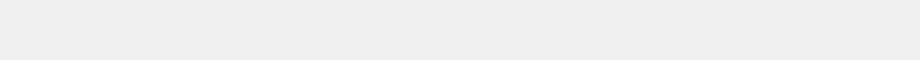Unique Numbers
Only Used With Floating Point Numbers
Unique Numbers
Minimum Value and Maximum Value sets the scaling range for the unique numbers list. These values can be any valid real number.
How Many Numbers is the Total count of numbers in the list to be scrambled. This is only used when generating floating point unique numbers. For Integers, this is set by the API as (Max-Min)+1). The maximum count of numbers in a list is 100,000.
How Many To Draw is the count of numbers returned from the scrambled list.
The Draw All Numbers option overrides this value.
How Many Columns arranges the numbers into the specified number of columns.
No HTML suppresses sending HTML wrapper codes. The HTML codes enable proper display of the numbers on a web browser. HTML codes are never used when the numbers are sent as a file.
Send as Text File sends the numbers as a text file instead of sending as a web page. If the total count of numbers is greater than 16384 then the numbers are always sent as a file.
Integers option selects to treat all numbers as integers.
Space Separated Columns have a single space characters between numbers in a row.
Comma Separated Columns
have a comma instead of a space character separating columns.
Quoted Comma Separated columns are separated by a comma and the numbers are surrounded by the double quotes character.
JSON Array returns the numbers with the following JSON format:

{"result":[
numbers here
]}

JSON Array Quoted Numbers is the same as JSON Array but each number is surrounded by the double quotes character.